Current in the circuit will be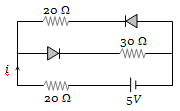(a) $\frac{5}{40}\mathrm{A}$
(b) $\frac{5}{50}\mathrm{A}$
(c) $\frac{5}{10}\mathrm{A}$
(d) $\frac{5}{20}\mathrm{A}$

Concept Questions :-

Applications of PN junction
High Yielding Test Series + Question Bank - NEET 2020

Difficulty Level:

The diode used in the circuit shown in the figure has a constant voltage drop of 0.5 V at all currents and a maximum power rating of 100 milliwatts. What should be the value of the resistor R, connected in series with the diode for obtaining maximum current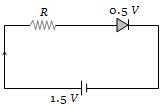(a) 1.5 $\mathrm{\Omega }$
(b) 5 $\mathrm{\Omega }$
(c) 6.67 $\mathrm{\Omega }$
(d) 200 $\mathrm{\Omega }$

Concept Questions :-

Applications of PN junction
High Yielding Test Series + Question Bank - NEET 2020

Difficulty Level:

In the following common emitter configuration an NPN transistor with current gain $\mathrm{\beta }$ = 100 is used. The output voltage of the amplifier will be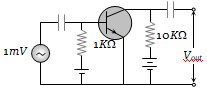(a) 10 mV
(b) 0.1 V
(c) 1.0 V
(d) 10 V

Concept Questions :-

Transistor
High Yielding Test Series + Question Bank - NEET 2020

Difficulty Level:

A sinusoidal voltage of peak value 200 volt is connected to a diode and resistor R in the circuit shown so that half wave rectification occurs. If the forward resistance of the diode is negligible compared to R the rms voltage (in volt) across R is approximately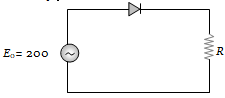(a) 200                            (b) 100
(c) $\frac{200}{\sqrt{2}}$                          (d) 280

Concept Questions :-

PN junction
High Yielding Test Series + Question Bank - NEET 2020

Difficulty Level:

The junction diode in the following circuit requires a minimum current of 1 mA to be above the knee point (0.7 V) of its I-V characteristic curve. The voltage across the diode is independent of current above the knee point. If ${\mathrm{V}}_{\mathrm{B}}$ = 5 V, then the maximum value of R so that the voltage is above the knee point, will be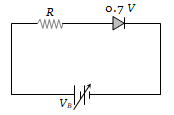(a) 4.3 k$\mathrm{\Omega }$                 (b) 860 k$\mathrm{\Omega }$

(c) 4.3 $\mathrm{\Omega }$                   (d) 860 $\mathrm{\Omega }$

Concept Questions :-

PN junction
High Yielding Test Series + Question Bank - NEET 2020

Difficulty Level:

In the circuit given below, V(t) is the sinusoidal voltage source, voltage drop ${\mathrm{V}}_{\mathrm{AB}}$(t) across the resistance R is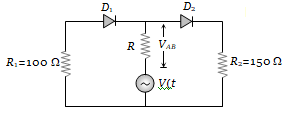(a) Is half wave rectified
(b) Is full wave rectified
(c) Has the same peak value in the positive and negative half cycles
(d) Has different peak values during positive and negative half cycle

Concept Questions :-

Applications of PN junction
High Yielding Test Series + Question Bank - NEET 2020

Difficulty Level:

The peak voltage in the output of a half-wave diode rectifier fed with a sinusoidal signal without filter is 10 V. The dc component of the output voltage is
(a)$10/\sqrt{2}$  V                                    (b) $10/\mathrm{\pi }$ V
(c) 10 V                                            (d) $20/\mathrm{\pi }$ V

Concept Questions :-

Rectifier
High Yielding Test Series + Question Bank - NEET 2020

Difficulty Level:

A transistor is used as an amplifier in CB mode with a load resistance of 5 k$\mathrm{\Omega }$. The current gain of the amplifier is 0.98 and the input resistance is 70 $\mathrm{\Omega }$, the voltage gain and power gain respectively are :
(a) 70, 68.6                          (b) 80, 75.6
(c) 60, 66.6                          (d) 90, 96.6

Concept Questions :-

Applications of Transistor
High Yielding Test Series + Question Bank - NEET 2020

Difficulty Level:

In the following circuits PN-junction diodes ${\mathrm{D}}_{1}$${\mathrm{D}}_{2}$ and ${\mathrm{D}}_{3}$ are ideal for the following potential of A and B, the correct increasing order of resistance between A and B will be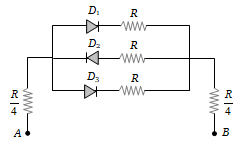(i) – 10 V, – 5V           (ii) – 5V, – 10 V
(iii) – 4V, – 12V

(a) (i) < (ii) < (iii)                       (b) (iii) < (ii) < (i)
(c) (ii) = (iii) < (i)                       (d) (i) = (iii) < (ii)

Concept Questions :-

Applications of PN junction
High Yielding Test Series + Question Bank - NEET 2020

Difficulty Level:

The circuit shown in the following figure contains two diode ${\mathrm{D}}_{1}$ and ${\mathrm{D}}_{2}$ each with a forward resistance of 50 ohms and with infinite backward resistance. If the battery voltage is 6 V, the current through the 100 ohm resistance (in amperes) is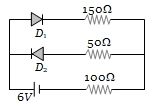(a) Zero                     (b) 0.02
(c) 0.03                     (d) 0.036

Concept Questions :-

PN junction